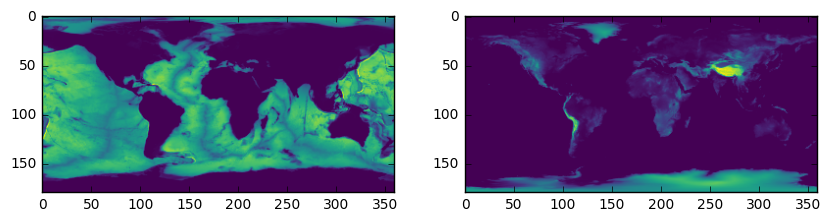# Multitaper Spectral Analysis Class Interface¶

The SHWindow class provides an interface to compute window functions that concentrate energy spatially into a given region and within a given spherical harmonic bandwidth. This allows to calculate spectral estimates localized to specific regions, and to generate a coupling matrix that couples degrees over a range that is as small as possible.

To demonstrate, in this example, we will determine the spectrum of the bathymetry of Earth and compare it with that of the surface topography. To this end, we will need to generate window functions that are separately concentrated over the land and the oceans. To start, we read the topography of Earth from the example files, create masks for the oceans and land, and plot the masks:

In :
%matplotlib inline
from __future__ import print_function # only necessary if using Python 2.x
import matplotlib.pyplot as plt
import numpy as np
from pyshtools.shclasses import SHCoeffs, SHGrid, SHWindow

infile = '../ExampleDataFiles/topo.dat.gz'
topo = np.loadtxt(infile)
nlat, nlon = topo.shape
lmax = nlat / 2 - 1
land_mask = (topo > 0)
ocean_mask = ~land_mask

fig, (col1, col2) = plt.subplots(1, 2, figsize=(13, 5))
col1.imshow(land_mask, extent=(-180, 180, -90, 90), cmap='viridis')
col1.set(xlabel='longitude', ylabel='latitude', title='land mask')
col2.imshow(ocean_mask, extent=(-180, 180, -90, 90), cmap='viridis')
col2.set(xlabel='longitude', ylabel='latitude', title='ocean mask');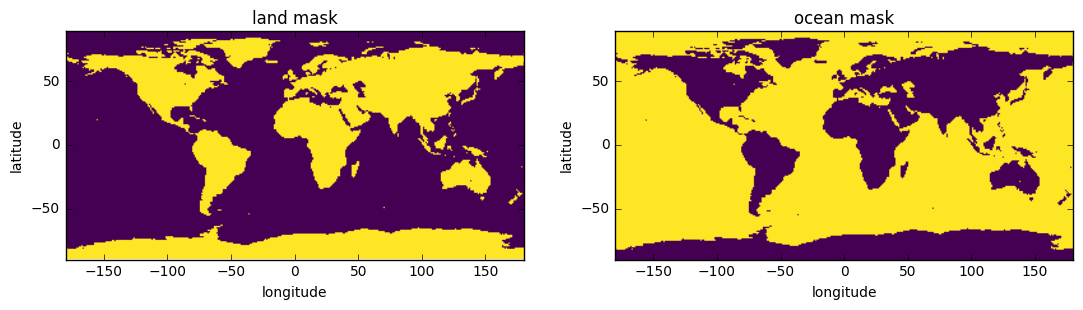## Optimally concentrated window functions¶

SHTOOLS provides a method to solve the so called concentration problem, that is, to find windows with a specified maximum spherical harmonic degree lmax that are optimally concentrated in the region of interest. The smaller the region, the larger lmax will need to be to allow for a good concentration. Because the oceans span a larger area than the land mass, lmax_ocean can therefore be smaller than lmax_land while still providing the same number of well concentrated windows.

Creating localization windows is easy using the SHWwindow method from_mask(). All we need to do is provide the mask, the maximum spherical harmonic bandwidth, and optionally, the number of windows to return:

In :
lmax_ocean = 7
lmax_land = 16
nwins = 10

ocean_windows = SHWindow.from_mask(ocean_mask, lmax_ocean, nwins)
land_windows = SHWindow.from_mask(land_mask, lmax_land, nwins)


We can find out information about the windows that were created using the info() method, and the windows can be plotted using the plot_windows() method. In plotting the windows, the concentration factor of the window is provided above each image. The concentration factor is simply the proportion of the energy of the window that lies within the region of interest, and it is seen that in order to obtain 10 well localized windows, the spectral bandwidth of the land windows needs to be more than twices as large as that of the ocean windows.

In :
print('ocean windows')
ocean_windows.info()
fig, ax = ocean_windows.plot_windows(nwins)

print('land windows')
land_windows.info()
fig, ax = land_windows.plot_windows(nwins)

ocean windows
kind = 'mask'
lwin = 7
nwin = 10
Taper weights are not set.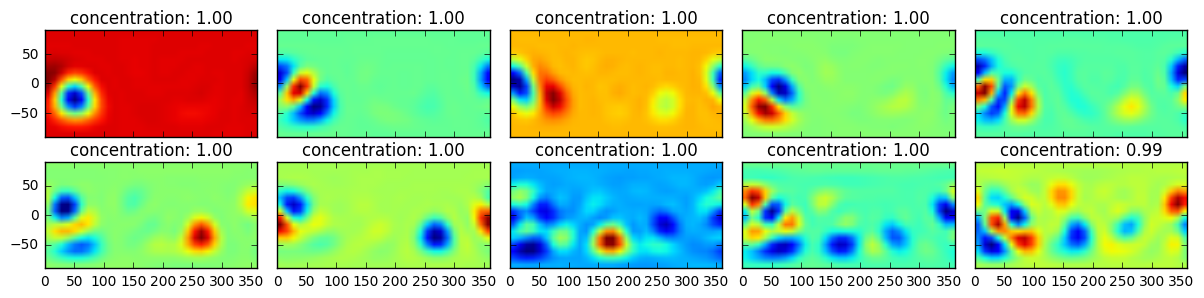land windows
kind = 'mask'
lwin = 16
nwin = 10
Taper weights are not set.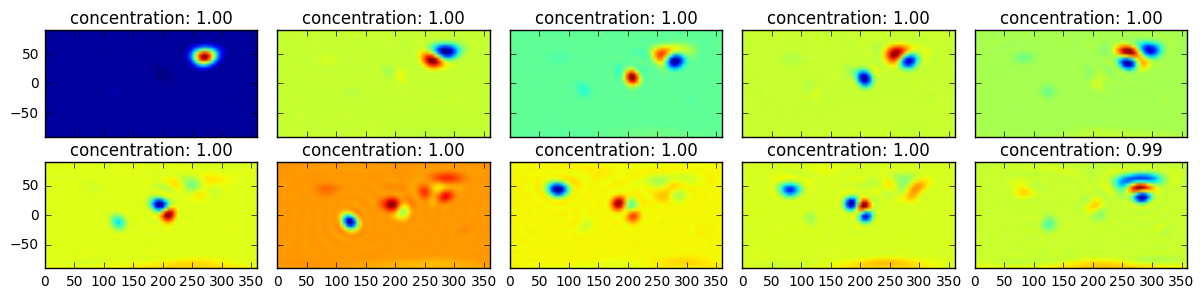The following plot shows the power spectra of all windows. In particular, it is noted that the power of the land windows extends to higher degrees than does the ocean windows. The ocean windows will thus allow for spectral estimates with lower biasses given the lower lmax of these windows.

In :
winpower_land = land_windows.spectra()
winpower_ocean = ocean_windows.spectra()

fig, ax = plt.subplots(1, 1)
for itaper in range(nwins):
ax.plot(winpower_ocean[:, itaper], c='blue')

for itaper in range(nwins):
ax.plot(winpower_land[:, itaper], c='green')

ax.set(xlabel='degree l', ylabel='power per degree l', title='ocean (blue) and land (green) window power');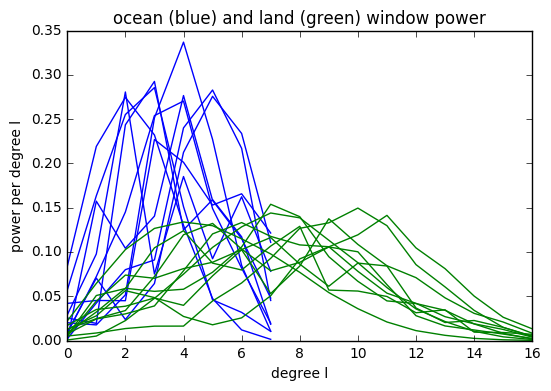## The coupling matrix¶

We next plot the coupling matrices associated with the land and ocean windows. For this example, we will assume that the topography of Earth is known only to degree 40.

In :
lmax_data = 40

lmax_valid1 = lmax_data - lmax_ocean
lmax_full1 = lmax_data + lmax_ocean
fig1, ax1 = ocean_windows.plot_coupling_matrix(lmax_data, nwins, mode='full', show=False)
ax1.plot([-0.5, lmax_data + 0.5], [lmax_valid1, lmax_valid1], c='red')
ax1.text(1, lmax_valid1 + 3, 'affected by degrees > lmax_data', fontsize=12, color='red')
ax1.set(xlim=[-0.5, lmax_data + 0.5], ylim=[lmax_full1, 0.], title='ocean coupling matrix')

fig2, ax2 = land_windows.plot_coupling_matrix(lmax_data, nwins, mode='full', show=False)
lmax_valid2 = lmax_data - lmax_land
lmax_full2 = lmax_data + lmax_land
ax2.plot([-0.5, lmax_data + 0.5], [lmax_valid2, lmax_valid2], c='red')
ax2.text(1, lmax_valid2 + 4, 'affected by degrees > lmax_data', fontsize=12, color='red')
ax2.set(xlim=[-0.5, lmax_data + 0.5], ylim=[lmax_full2, 0.], title='land coupling matrix');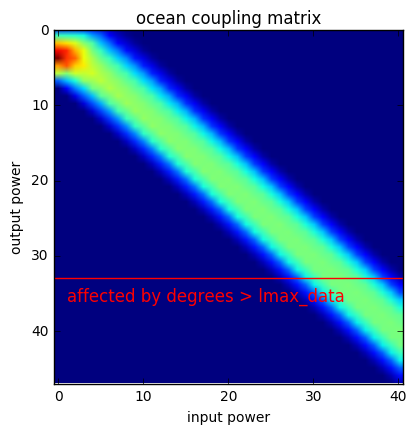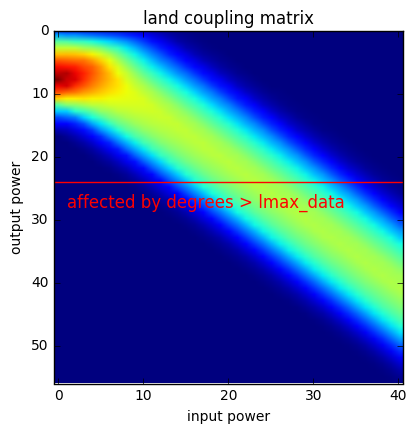The coupling matrix reflects the different quality of the land and ocean spectra. The higher degree part (towards the bottom right) corresponds to a simple convolution, as it becomes asymptotically symmetric about the diagonal. The land windows (bottom) couple degrees over a wider range and therefore decrease the spectral resolution that can be achieved.

It is also seen that the coupling matrix is asymmetric at the lowest degrees. Here, low input degrees map to higher output degrees. For example, the input degree 0 maps strongest to about degree 3 in the ocean case and to about degree 8 in the land case. This is a consequence of the fact that we can not estimate the global mean or very large wavelength structures from subregions that are much smaller than the feature of interest. Low degree energy maps therefore to the lowest window degrees, where they increase the overall power.

A given localized spectral estimate at degree l contains information from the global field between degrees l-lwin and l+lwin. For this example, we assumed that the data were expanded to degree 40. The red line in these plots thus denotes the degree where the localized spectral esimates contain information from beyond the maximum degree of the data. In general, one should only interpret the localized spectrum up to a maximum degree lmax_data - lwin.

### Regional spectral estimates¶

We are now in a position to compute regional power spectral estimates of the land and of the ocean topography as well as the corresponding power spectra that we would expect to see on land and in the ocean if Earth's topography was stationary and isotropic.

We first expand the topography into spherical harmonics, and calculate the global power spectrum:

In :
grid_topo = SHGrid.from_array(topo)
coeffs_topo = grid_topo.expand()

power_global = coeffs_topo.spectrum()
lmax_global = coeffs_topo.lmax
ls_global = np.arange(len(power_global))


Next, we use the methods multitaper_spectrum() to determine the multitaper power spectrum of the oceans and land, and then we calculate the expected spectrum if the Earth's topography was stationary and isostropic. For the later, we use the dot product of the coupling matrix and global spectrum, but we also could have used the method biased_spectrum():

In :
power_ocean, dpower_ocean = ocean_windows.multitaper_spectrum(coeffs_topo, nwins)
power_land, dpower_land = land_windows.multitaper_spectrum(coeffs_topo, nwins)

cmatrix_ocean = ocean_windows.coupling_matrix(lmax_global, nwins, mode='valid')
power_ocean_predicted = np.dot(cmatrix_ocean, power_global)
ls_ocean = np.arange(len(power_ocean))

cmatrix_land = land_windows.coupling_matrix(lmax_global, nwins, mode='valid')
power_land_predicted = np.dot(cmatrix_land, power_global)
ls_land = np.arange(len(power_land))


Finally, let's plot the masked topography of the oceans and land, and then plot the measured and predicted multitaper power spectra:

In :
fig, (col1, col2) = plt.subplots(1, 2, figsize=(10, 5))
col1.imshow(-topo * ocean_mask, cmap='viridis')
col2.imshow(topo * land_mask, cmap='viridis')

fig, ax = plt.subplots(1, 1, figsize=(10, 5))
ax.plot(ls_global, power_global, c='grey', alpha=0.5, lw=2, label='global')
ax.plot(ls_ocean, power_ocean, '-', c='blue', label='ocean (measured)')
ax.plot(ls_ocean, power_ocean_predicted, '--', c='blue', label='ocean (predicted)')
ax.plot(ls_land, power_land, '-', c='green', label='land (measured)')
ax.plot(ls_land, power_land_predicted, '--', c='green', label='land (predicted)')

ax.legend(loc=3)
ax.set(xscale='log', yscale='log', xlabel='degree', ylabel='power')
ax.grid(True)